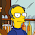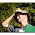## Monday, May 11, 2015

### Five Years of "The Search for a Search"

The Journal of Advanced Computational Intelligence and Intelligent Informatics published the paper The Search for a Search: Measuring the Information Cost of Higher Level Search of William A. Dembski and Robert J. Marks II (DM) in its July edition in 2010. With the five year jubilee of the publication coming, it seems to be appropriate to revisit a pet peeve of mine...

(Shell game performed on Karl-Liebknecht-Straße in Berlin, photograph by E.asphys)

Imagine a shell game. You have observed the con artist for a while, and now you know:

1. The pea ends up under each of the three shells (left, middle, and right) with the same probability, i.e., $$P(Pea=left)=P(Pea=middle)=P(Pea=right)=1/3$$
2. If the pea ends up under the left or the middle shell, you are able to track its way. So, in these cases, you will find the pea with probability 1 $$P(Finding\,Pea|Pea=left)=P(Finding\,Pea|Pea=middle)=1$$
3. However, if the pea ends up under the right shell, in 999 times out 1000, you make a mistake during your tracking and be convinced that it is under the left or the middle shell - the probability of finding this pea is 1/1000$$P(Finding\,Pea|Pea=right)=1/1000$$

You are invited to play the game. Should you use your knowledge (method $M_1$), or should you chose a shell at random (method $M_2)$? Let's calculate the average probability for finding the pea using your knowledge $$AM_1= P(Pea=left) \cdot P(Finding\,Pea|Pea=left)$$ $$+ P(Pea=middle) \cdot P(Finding\,Pea|Pea=middle)$$ $$+ P(Pea=right) \cdot P(Finding\,Pea|Pea=right)$$ $$AM_1 = \frac{1}{3} \cdot 1 + \frac{1}{3} \cdot 1 + \frac{1}{3} \cdot \frac{1}{1000} = \frac{2001}{3000} \approx \frac{2}{3}$$

What is the average probability when choosing a shell at random? As the pea is in the left, middle or right position with the same probability, we get $$AM_2 = \frac{1}{3}$$

So, $M_1$ or $M_2$? The answer seem to be obvious: you should stick to the first method, as it wins twice as often. Not so fast, say the Drs Dembski and Marks. You should calculate the average of the active information - the active entropy: $$H_1 = \frac{1}{3} \cdot \log_2 \frac{1}{1/3} + \frac{1}{3} \cdot \log_2 \frac{1}{1/3} +\frac{1}{3} \cdot \log_2 \frac{1/1000}{1/3}$$ $$= \log_2(\sqrt{1 \cdot 1 \cdot \frac{1}{1000}}) - \log_2(\sqrt{\frac{1}{3}\cdot \frac{1}{3} \cdot \frac{1}{3}})$$ $$=-\log_2(10) + \log_2(3)\approx -1.737$$ And their conclusion (p. 477): as on average the calculation results in negative active information, the search performance is rendered worse than random search. This is obviously false.

What went wrong? To calculate the overall performance, it is appropriate to use the arithmetic mean, as done for $AM_1$ and $AM_2$. By calculating the active entropy, you are de facto comparing the geometric means of your method and of the random method. The geometric mean favors equidistribution, thereby preferring the inferior random method over your more successful method, which tends to fail in only one case (pea under the third shell). This is a usual scenario: you often find algorithms which do well on most of the cases, but fail in some - often especially manufactured - instances.

Conclusion: The average of the active information is not a good method to describe the performance of a search. If you think otherwise, maybe I can interest you in buying a bridge?

(edited to clarify the search process)

1.I hope you don't mind my observation that your post relates to one of three questions you posed at Ask Dr Ewert (link expires June 30, 2015). Ewert, who collaborates with Dembski and Marks, evidently intends to answer selected questions at Uncommon Descent. You've been banned there since raising the questions, have you not? Correlation does not imply causation. But if you cannot comment on his answers to your questions, then he will in fact have ensconced them in a sham forum.

1.2.I hadn't thought about where Winston Ewert would post his answers, but Uncommon Descent seems to be an obvious choice. Though it is not ideal, Winston is at least trying to talk to "the other side"!

My banning was just coincidental (though perhaps convenient): I asked three times about the disappearing comments of Aurelio Smith, and only after I got banned I realized that it wasn't a technical problem that my questions vanished quickly!

2.I don’t think it is particularly relevant to your general point but I think your sums could be wrong. The correct answer depends on several things which need to be defined more closely. What counts as a successful search? What do you mean when you say the probability of finding the pea is 1/1000? What do you know? What are the searches you are comparing? Here are some of the options as I see them.

What counts as success?

Is it choosing the shell which contains the pea

OR

Choosing the correct shell and finding the pea i.e. choosing correctly and knowing you have chosen correctly.

Meaning of probability of finding the pea is 1/1000

Do you mean the probability of finding the pea after you have chosen the shell and it is turned over (kind of weird but conceivable)? Or do you mean if the pea goes under that shell what is the probability of you seeing it happen?

What you know

Suppose the shells are A, B and C.

1) You know that if the pea is under A or B you will see it there. (In which case the logical strategy is if you see the pea under A or B then choose A or B otherwise choose C. Probability of success is 100%. I guess this is not what you meant.)

OR

2) You know that for two of the shells (but you don’t know which ones) if the pea is under them you will see it there.

3) You know nothing in advance but learn something if the pea is under shells A or B.

Practically speaking 2 and 3 come to the same thing.

Search strategies

In the case of ( 2) and ( 3) I guess the two possible search strategies are:

X) Choose a shell at random (even if you can see it is under another one!)

Y) If you can see the pea under a shell choose that one, otherwise choose a shell at random

Calculation of Probabilities

If success is choosing the correct shell

The P(success) for X is 1/3 and P(success) for Y is 7/9 (1/3*1 + 1/3*1 + 1/3*1/3)

If success is finding the pea & p(1/1000) means probability of seeing the pea go under the shell then

P(success) for X is 1/3 and P(success) for Y is 7/9 – same argument as above

If success is finding the pea & p(1/1000) means probability of finding the pea once it is turned over

P(success) for X 2/9 (1/3*1/3 + 1/3*1/3 + 1/3*1/3*1/1000) and P(success) for Y is 2/3

1.I'm afraid I cannot follow. I edited my post to clarify my position: it is just a traditional shell game ("Hütchenspiel"). If you correctly announce the shell under which there is the pea, you win - you have performed a successful search.

3.Hi - I thought I made one short response that has not appeared but maybe it did not take. I think I have got it now.

Strategy M1 is: try as hard as you can to track the pea and choose the shell you think it is under (which as it turns out with these probabilities will mean you almost always choose left or middle).

Strategy M2: is pick a shell at random.

M1 is better because two of the shells have a high correlation between appearing to have the pea and actually having the pea.